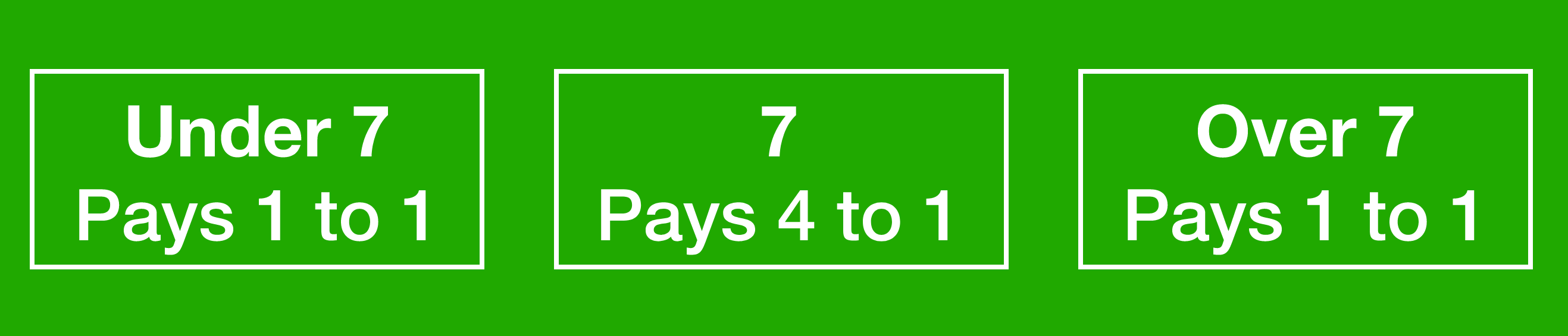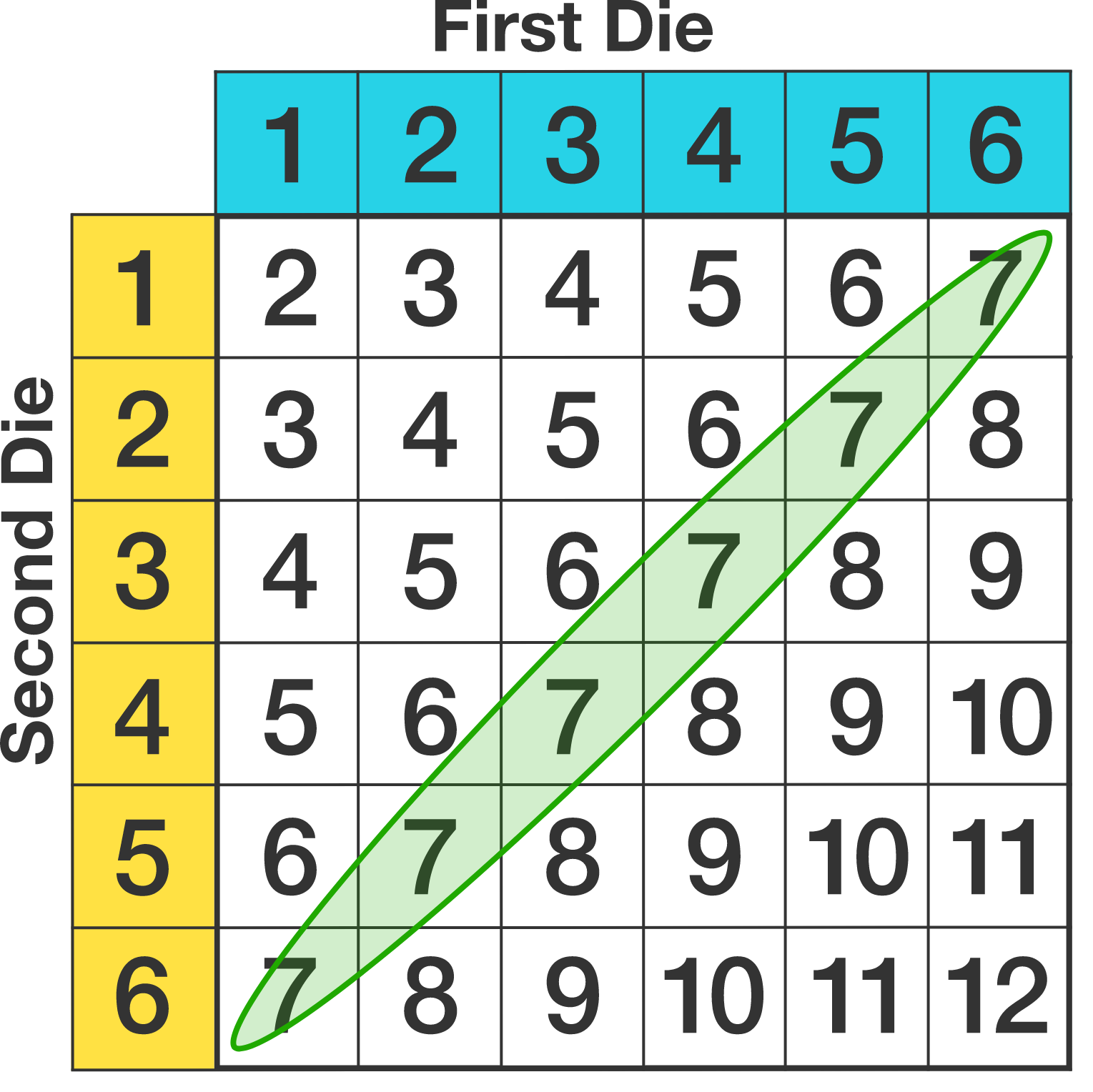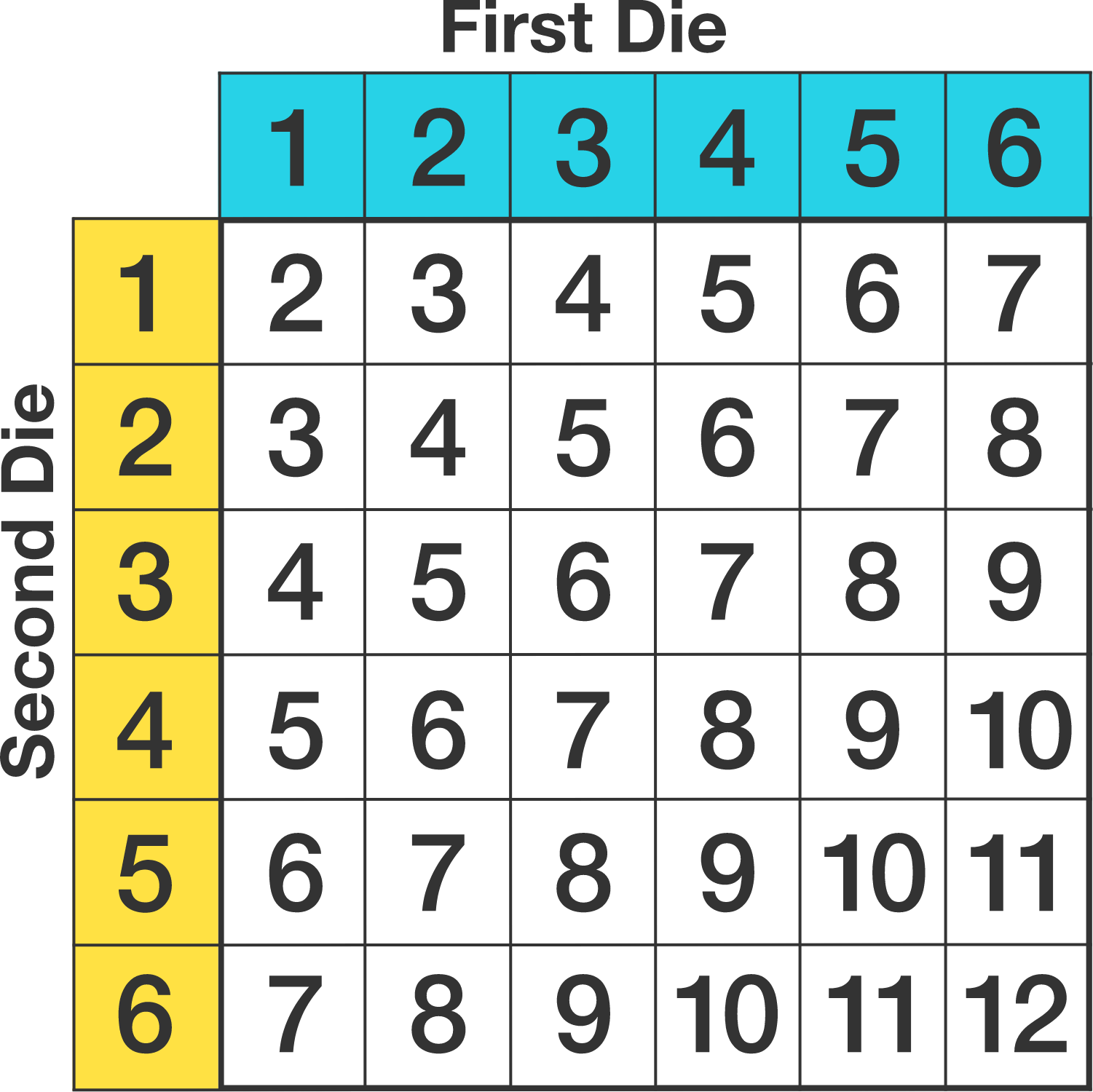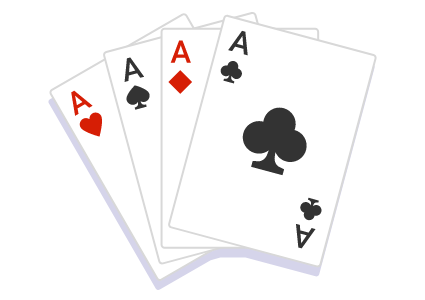### Casino Probability

For this last set of introductory questions, we're going to analyze two dice games; both have been played in real casinos.

For the first game, Under and Over, there are three possible bets:The player picks a square and bets however much they want, and then rolls two dice. They win on Under $7$ if they roll $\textbf2, \textbf3, \textbf4, \textbf5,$ or $\textbf6$. They win on Over $7$ if they roll $\textbf8, \textbf9, \textbf1\textbf0, \textbf1\textbf1,$ or $\textbf1\textbf2$. On $7,$ they only win if they roll exactly 7.

There is a $\frac{6}{36} = \frac{1}{6}$ probability of rolling a $7$ on a pair (count on the grid below). What is true about the probabilities of Over and Under?# Avoid the Scam

In casino bets, the most likely to win isn't necessarily the best, because bets don't all pay equally:With Under and Over, a bet of $\100$ on Under or Over wins back $\100$ (no profit); a bet of $\100$ on $7$ wins $\400$ $($and the player keeps the $\100$ that they bet$).$

You can use the expected value to compare the outcomes. Which square is best to bet on?# Avoid the ScamAs found from the solution in the last question, assuming a bet of $100 per turn, the player has an expected value of $\83.33$ per turn no matter which square they bet on. This means the casino is earning $\100 - \83.33 = \16.67$ per turn. It is said to have a house advantage of $16.67\%.$ $($That is, whatever you bet, you can suppose in the long run the casino takes $16.67\%$ of it per turn.$)$ $16.67\%$ is an extremely high house advantage for a casino game; Over and Under has been called "one of the biggest sucker bets to come down the pike" and is essentially no longer played today. $($A game called Keno can be worse, with a house advantage roughly around $15\%$ to $30\%$ depending on the bet, although it is played at a much slower pace so it won't drain the gambler's pocket as quickly.$)$ Knowing the house advantage for each casino game lets us compare their quality and if they're worth playing. We'll try another house advantage calculation, this time with a game that you can still sometimes find in modern casinos: simplified craps. # Avoid the Scam Simplified Craps does not involve any player choice, other than the amount we want to bet, but our goal is to figure out the house advantage; is this a good game to play, or a "sucker bet"? The player bets however much they want, rolls two dice, and wins if they throw a $\textbf{2, 3, 4, 10, 11,}$ or $\textbf{12}$; otherwise they lose their bet (and the casino wins). Just like with Over and Under, not every type of bet earns the same amount of money; but before getting to that part of the rules, who is more likely to win on a particular roll, the player or the casino?# Avoid the Scam These are the payouts for Simplified Craps:  Rolling Payout $2$ $3$ to $1$ $3,$ $4$ $1$ to $1$ $10,11$ $2$ to $1$ $12$ $5$ to $1$ This means, for example, a bet of $\100$ with a roll of $12$ wins the player back $\500$ plus the amount of their bet. A roll of $3$ or $4$ on a bet of $\100$ wins$100 $($and also gets back the amount of the bet $\100).$ The player loses their bet if the roll comes up $5,$ $6,$ $7,$ $8,$ or $9.$

We want to find the expected value for the game, supposing a player who bets $\100$ each turn. Which of these calculations is correct?Note: If a player loses their money on a particular turn, they end the round with $\0,$ not a negative amount of money.

# Avoid the Scam

In the previous calculation, we found that betting $\100$ every turn leads to an expected value to the player of $\97.22.$

What is the house advantage? (That is, in the long run, what percent of each dollar spent on the game does the casino get?)

# Avoid the Scam

To compare our two games, we had a house advantage of $16.67\%$ vs. one of $2.78\%.$ It means, in essence, Over and Under will earn money $6$ times faster for the casino than Simplified Craps. $(2.78\%$ is generally considered reasonable by gamblers, although there are games where the house advantage is even smaller$.)$

With both these games, there weren't any choices that mattered, but some casino games involve a wide variety of bets that not only need to be analyzed separately but can change the house advantage. This course will consider situations in Blackjack, Craps, and Poker, and get you practice in finding the best way to win!×